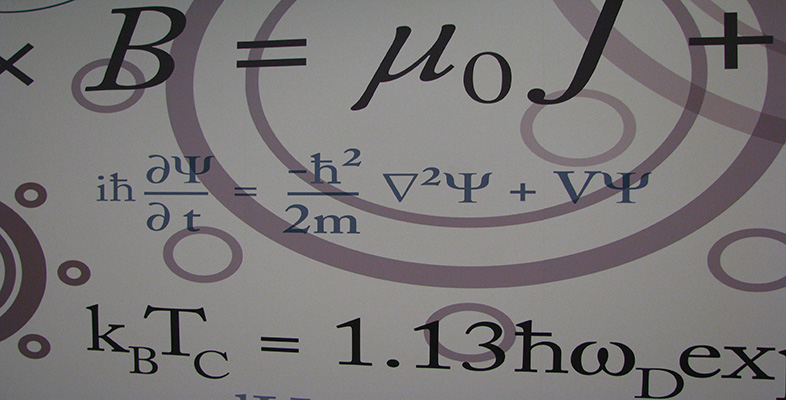Number systems

This free course is available to start right now. Review the full course description and key learning outcomes and create an account and enrol if you want a free statement of participation.

Free course

1.3 Further exercises

Exercise 4

Solve the following linear equations.

• (a)   5x + 8 = −2

• (b)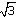x + 2 = −5

• (c)  2x −1 = 5

• (d)  5x + 4 = 3

State in each case whether the solution belongs to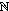,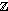,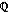and/or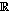.

Solution

• (a)  x = −2, which belongs to(and hence toand).

• (b)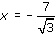, which belongs to.

• (c)  x = 3, which belongs to(and hence to,and).

• (d)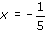, which belongs to(and hence to).

Remark Each linear equation with coefficients inhas a solution in(because the equation ax + b = 0 where a, b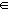and a ≠ 0 has solution x = − b/a, which involves only additive inverses and division of rational numbers). Similarly each linear equation with coefficients inhas a solution in. The same is not true of, as part (d) illustrates.

Exercise 5

Show that there is no rational number x such that x2 = 3.

Solution

Suppose there is a rational number x such that x2 = 3. Then we can write x = p/q, where p and q are positive integers whose greatest common factor is 1.

Then the equation x2 = 3 becomes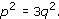Now p is either divisible by 3 or has remainder 1 or 2 on division by 3;

that is, p = 3k or 3k + 1 or 3k + 2 for some integer k.

But if p = 3k + 1, then p2 = 9k2 + 6k + 1 is not divisible by 3, and if p = 3k + 2, then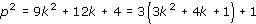is not divisible by 3. So, since 3q2 is divisible by 3, we conclude that p = 3k.

Hence (3k)2 = 3q2, so q2 = 3k2.

But then the same argument applies to q to show that q must also be divisible by 3.

Hence 3 is a common factor of p and q.

This is a contradiction, so we conclude that the assumption must have been false. Hence there is no rational number x such that x2 = 3.

M208_6